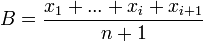## Wednesday, September 30, 2009

### Fast average calculation for website

In this article, I will explain how to efficiently calculate the average user rating of a zillion post or more generally how to calculate an average value when data comes at interval and the average need to be recalculated every time.

The arithmetic mean is calculated using the following formula :If we add another value the formula becomes :The interesting part is how we can calculate the new value B from the last average value A :We need 3 values to calculate the new average : the last average, the last count of values contained in the last average and a new value to be considered. Put simply, we need to multiply the last average by the number of value in it and add the new value to be counted in the average. Then we divide the result by the new number of value in the average.

This is good enough method to calculate an average. It's a bit less precise than the usual method because of rounding error, but it's still much more precise than needed for any website use. Off course, you would need to store the average and the value count in a DB.